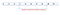# Minimum Operations to Make Array Equal: Understanding the Thought Process for Interviews and Leetcoding

This is a standard algorithmic problem confirmed to have been asked in many technical interviews. This problem appeared in the April 2021 Leetcode monthly challenge with the difficulty of Medium. Whether you are prepping for a technical interview or you are just an algorithm lover like me, I strongly believe this post will make an interesting read.

Problem Statement You have an array `arr` of length `n` where `arr[i] = (2 * i) + 1` for all valid values of `i` (i.e. `0 <= i < n`). In one operation, you can select two indices `x` and `y` where `0…`

# Shortest Unsorted Continuous Subarray

In this post, I will be explaining my solution to one of the Leetcode algorithmic problems for February 2021 monthly challenge.Shortest Unsorted Continuous Subarray

PROBLEM STATEMENT
Given an integer array `nums`, you need to find one continuous subarray that if you only sort this subarray in ascending order, then the whole array will be sorted in ascending order. Return the shortest such subarray and output its length.

SOLUTION As can be seen from the above image, a Shortest Continuous Subarray is the shortest unsorted subarray of an array that when sorted would leave the whole array sorted in that order. For example, in…

# How to Convert Roman Numerals to Integer

In this post, I will be solving one of the interesting and easy algorithmic questions that appeared in the February 2021 Leetcode Monthly challenge.

PROBLEM STATEMENT
Given the following Roman symbols and their integer representations, write an algorithm to convert Roman Numerals to Integer;

`Symbol       ValueI             1V             5X             10L             50C             100D             500M             1000`

Few Explanatory Points Roman numerals are represented by the 7 symbols in the above table and are usually written largest to smallest from left to right. For example, `2` is written as `II` in Roman numeral, just two ones' added…

# Find the Location of the Max Element in an Unsorted Array Using Binary Search

In this post, I will be explaining a solution to one of the most interesting algorithmic problems I encountered recently. At the same time, demystify a common misconception about BINARY SEARCH (BS).

PROBLEM STATEMENT There is an unsorted array of size n ( 2 ≤ n ≤ 10⁵). The array contains distinct integer values. You are given the size of the array n and your task is to find the index ( 1 ≤index≤n) of the largest element in the array. You are also given access to an object from which you can query the position of the second maximum…## Emmanuel Wilson

Software Engineer | Competitive Programmer | Graduate Student in SE & Data Analysis | Music freak :-) I write about tech and problem solving